Anúncio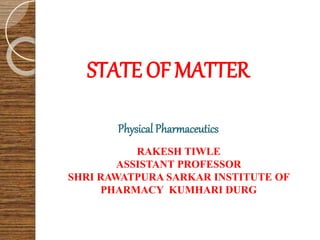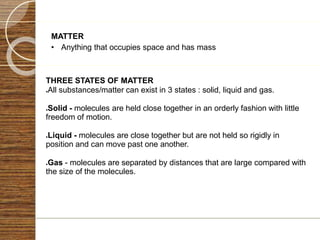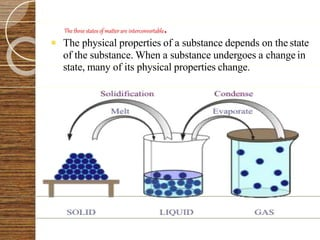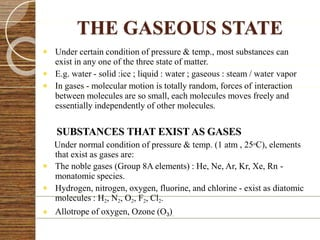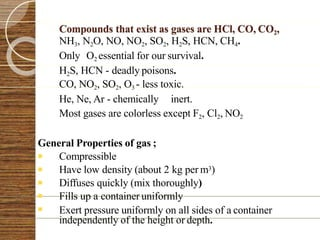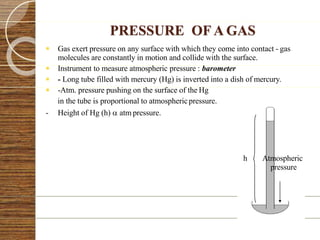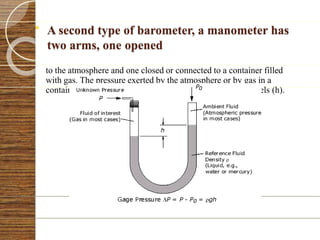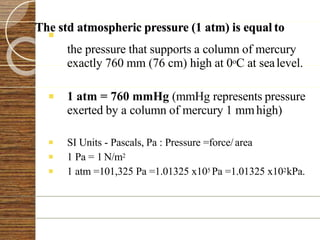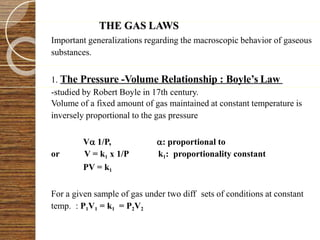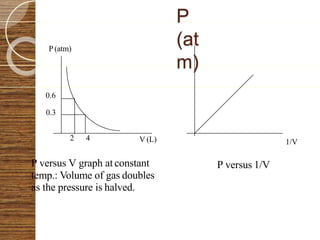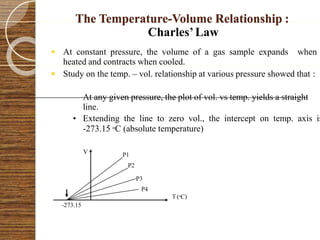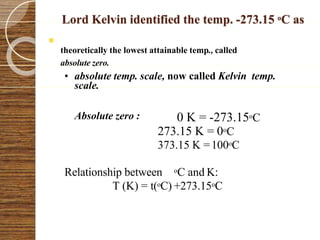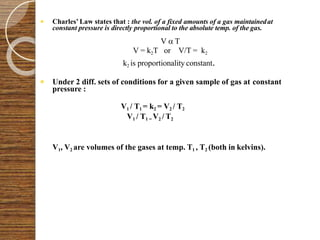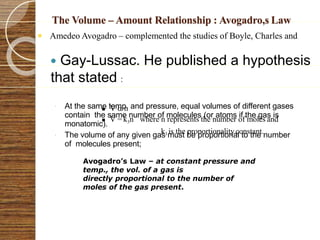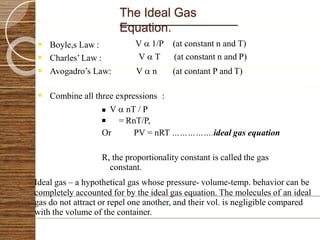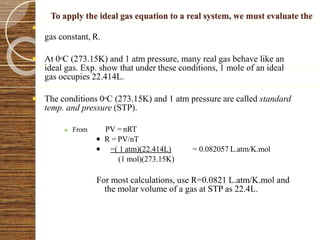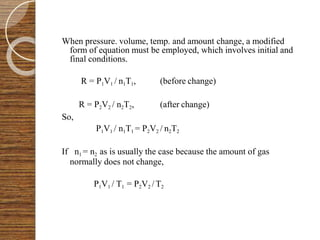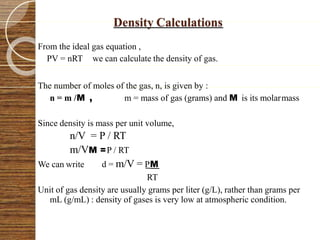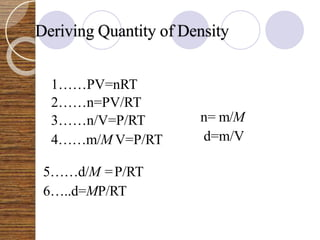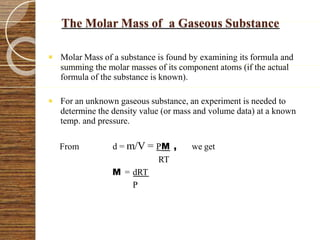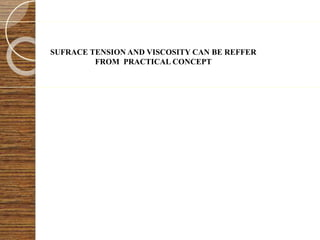1 de 22
Anúncio

### State of matter

1. STATE OFMATTER Physical Pharmaceutics RAKESH TIWLE ASSISTANT PROFESSOR SHRI RAWATPURA SARKAR INSTITUTE OF PHARMACY KUMHARI DURG
2. MATTER • Anything that occupies space and has mass THREE STATES OF MATTER ●All substances/matter can exist in 3 states : solid, liquid and gas. ●Solid - molecules are held close together in an orderly fashion with little freedom of motion. ●Liquid - molecules are close together but are not held so rigidly in position and can move past one another. ●Gas - molecules are separated by distances that are large compared with the size of the molecules.
3. The three statesof matter are interconvertable.  The physical properties of a substance depends on the state of the substance. When a substance undergoes a change in state, many of its physical properties change.
4. THE GASEOUS STATE  Under certain condition of pressure & temp., most substances can exist in any one of the three state of matter.  E.g. water - solid :ice ; liquid : water ; gaseous : steam / water vapor  In gases - molecular motion is totally random, forces of interaction between molecules are so small, each molecules moves freely and essentially independently of other molecules. SUBSTANCES THAT EXIST AS GASES Under normal condition of pressure & temp. (1 atm , 25oC), elements that exist as gases are:  The noble gases (Group 8A elements) : He, Ne, Ar, Kr, Xe, Rn - monatomic species.  Hydrogen, nitrogen, oxygen, fluorine, and chlorine - exist as diatomic molecules : H2, N2, O2, F2, Cl2.  Allotrope of oxygen, Ozone (O3).
5. Compounds that exist as gases are HCl, CO, CO2, NH3, N2O, NO, NO2, SO2, H2S, HCN, CH4. Only O2 essential for our survival. H2S, HCN - deadly poisons. CO, NO2, SO2, O3 - less toxic. He, Ne, Ar - chemically inert. Most gases are colorless except F2, Cl2, NO2 General Properties of gas ;  Compressible  Have low density (about 2 kg per m3)  Diffuses quickly (mix thoroughly)  Fills up a container uniformly  Exert pressure uniformly on all sides of a container independently of the height or depth.
6. PRESSURE OF A GAS  Gas exert pressure on any surface with which they come into contact - gas molecules are constantly in motion and collide with the surface.  Instrument to measure atmospheric pressure : barometer  - Long tube filled with mercury (Hg) is inverted into a dish of mercury.  -Atm. pressure pushing on the surface of the Hg in the tube is proportional to atmosphericpressure. - Height of Hg (h)  atm pressure. h Atmospheric pressure
7.  A second type of barometer, a manometer has two arms, one opened to the atmosphere and one closed or connected to a container filled with gas. The pressure exerted by the atmosphere or by gas in a container is proportional to the difference in the mercury levels (h).
8.  The std atmospheric pressure (1 atm) is equal to the pressure that supports a column of mercury exactly 760 mm (76 cm) high at 0oC at sealevel.  1 atm = 760 mmHg (mmHg represents pressure exerted by a column of mercury 1 mmhigh)  SI Units - Pascals, Pa : Pressure =force/area  1 Pa = 1 N/m2  1 atm =101,325 Pa =1.01325 x105 Pa =1.01325 x102kPa.
9. THE GAS LAWS Important generalizations regarding the macroscopic behavior of gaseous substances. 1. The Pressure -Volume Relationship : Boyle’s Law -studied by Robert Boyle in 17th century. Volume of a fixed amount of gas maintained at constant temperature is inversely proportional to the gas pressure V 1/P, : proportional to or V = k1 x 1/P k1: proportionality constant PV = k1 For a given sample of gas under two diff sets of conditions at constant temp. : P1V1 = k1 = P2V2
10. P (atm) 1/V 0.6 0.3 2 4 P versus V graph at constant temp.: Volume of gas doubles as the pressure is halved. P (at m) V (L) P versus 1/V
11. The Temperature-Volume Relationship : Charles’Law  At constant pressure, the volume of a gas sample expands when heated and contracts when cooled.  Study on the temp. – vol. relationship at various pressure showed that : At any given pressure, the plot of vol. vs temp. yields a straight line. • Extending the line to zero vol., the intercept on temp. axis is -273.15 oC (absolute temperature) V -273.15 P1 P2 P3 P4 T (oC)
12.  Lord Kelvin identified the temp. -273.15 oC as theoretically the lowest attainable temp., called absolute zero. • absolute temp. scale, now called Kelvin temp. scale. Absolute zero : 0 K = -273.15oC 273.15 K = 0oC 373.15 K =100oC Relationship between oC and K: T (K) = t(oC) +273.15oC
13.  Charles’ Law states that : the vol. of a fixed amounts of a gas maintainedat  Under 2 diff. sets of conditions for a given sample of gas at constant pressure : V1 / T1 = k2 = V2 / T2 V1 / T1 = V2 / T2 V1, V2 are volumes of the gases at temp. T1 , T2 (both in kelvins). constant pressure is directly proportional to the absolute temp. of the gas. V  T V = k2T or V/T = k2 k2 is proportionality constant.
14. The Volume – Amount Relationship : Avogadro,s Law  Gay-Lussac. He published a hypothesis that stated :  At the same temp. and pressure, equal volumes of different gases contain the same number of molecules (or atoms if the gas is monatomic).  The volume of any given gas must be proportional to the number of molecules present;  Amedeo Avogadro – complemented the studies of Boyle, Charles and  V  n  V = k3n where n represents the number of moles and k3 is the proportionalityconstant. Avogadro’s Law – at constant pressure and temp., the vol. of a gas is directly proportional to the number of moles of the gas present.
15. The Ideal Gas Equation.  Boyle,s Law :  Charles’ Law : V  1/P (at constant n and T) V  T (at constant n and P)   Avogadro’s Law: V  n (at contant P and T) Combine all three expressions :  V  nT / P  = RnT/P, Or PV = nRT …………….ideal gas equation R, the proportionality constant is called the gas constant. Ideal gas – a hypothetical gas whose pressure- volume-temp. behavior can be completely accounted for by the ideal gas equation. The molecules of an ideal gas do not attract or repel one another, and their vol. is negligible compared with the volume of the container.
16.  To apply the ideal gas equation to a real system, we must evaluate the gas constant, R.  At 0oC (273.15K) and 1 atm pressure, many real gas behave like an ideal gas. Exp. show that under these conditions, 1 mole of an ideal gas occupies 22.414L.  The conditions 0oC (273.15K) and 1 atm pressure are called standard temp. and pressure (STP).  From PV = nRT  R = PV/nT  =( 1 atm)(22.414L) = 0.082057 L.atm/K.mol (1 mol)(273.15K) For most calculations, use R=0.0821 L.atm/K.mol and the molar volume of a gas at STP as 22.4L.
17. When pressure. volume, temp. and amount change, a modified form of equation must be employed, which involves initial and final conditions. R = P1V1 / n1T1, (before change) So, R = P2V2 / n2T2, (after change) P1V1 / n1T1 = P2V2 / n2T2 If n1 = n2 as is usually the case because the amount of gas normally does not change, P1V1 / T1 = P2V2 / T2
18. Density Calculations From the ideal gas equation , PV = nRT we can calculate the density of gas. The number of moles of the gas, n, is given by : n = m /M , m = mass of gas (grams) and M is its molarmass Since density is mass per unit volume, n/V = P / RT m/VM =P / RT We can write d = m/V = PM RT Unit of gas density are usually grams per liter (g/L), rather than grams per mL (g/mL) : density of gases is very low at atmospheric condition.
19. Deriving Quantity of Density 1……PV=nRT 2……n=PV/RT 3……n/V=P/RT 4……m/M V=P/RT n= m/M d=m/V 5……d/M =P/RT 6…..d=MP/RT
20. The Molar Mass of a Gaseous Substance  Molar Mass of a substance is found by examining its formula and summing the molar masses of its component atoms (if the actual formula of the substance is known).  For an unknown gaseous substance, an experiment is needed to determine the density value (or mass and volume data) at a known temp. and pressure. From d = m/V = PM , we get RT M = dRT P
21. SUFRACE TENSION AND VISCOSITY CAN BE REFFER FROM PRACTICAL CONCEPT
22. THANK YOU
Anúncio